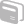# Activities

••• ##### Subject Area

• Math: Algebra I: Expressions
• Math: Algebra I: Equations

• ##### Author6-8

60 Minutes

• ##### Device
•TI-Nspire™ CX/CX II
•TI-Nspire™ CX CAS/CX II CAS
• TI-Nspire™
• TI-Nspire™ CAS
• ##### Software

TI-Nspire™
TI-Nspire™ CAS

3.1

## TI-PoW: Chirp! Chirp! - I Notice, I Wonder

#### Activity Overview

This problem presents an opportunity for students to think about patterns, units of measurement and also to work on expressing the pattern as a formula that will lead them to thinking algebraically. If your state has adopted the Common Core State Standards, this alignment might be helpful: Grade 6: Expressions & Equations 6.EE.6. Use variables to represent numbers and write expressions when solving a real-world or mathematical problem. Grade 7: Expressions & Equations Solve real-life and mathematical problems using numerical and algebraic expressions and equations. Grade 8: Expressions & Equations 8.EE.7. Solve linear equations in one variable.